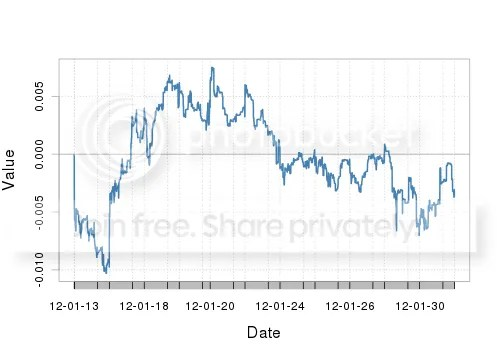Want to share your content on R-bloggers? click here if you have a blog, or here if you don't.

While ago I built two R scripts to track OMX Baltic Benchmark Fund against the index. One script returns the deviation of  fund from the index and it works fast enough. The second calculates the value of the fund every minute and it used to take for while. For example, it spent 2 minutes or more to get the values for one day. Here is an example of the result:Following piece of code was in question:

?View Code RSPLUS
 ```for(y in 1:NROW(x)) { z=x[y,] if(as.numeric(z\$last_price>0)) { if(as.numeric(z\$bid>z\$last_price))rez[y]=z\$bid else if(as.numeric(z\$ask>0 & z\$ask

The code above loops over time series and based on set of rules tries to decide which price (bid, ask or previous one) to use for calculations. Pure R script used to take 100 seconds to derive the price.

During the weekend I found time to watch very interesting Rcpp presentation. To my surprise, there are numerous ways to seamlessly integrate C++ into R code. So, I decided to rewrite the code above in C++ (Rcpp and inline packages were used).

?View Code RSPLUS
 ```#c++ code embed in code value code=' NumericVector bid(bid_);NumericVector ask(ask_);NumericVector close(close_);NumericVector ret(ask_); int bid_size = bid.size(); for(int i =0;i0) { if(bid[i]>close[i]) { ret[i] = bid[i]; } else if(ask[i]>0 && ask[i]

What did I get in return? Well, 0.1 of a second instead of 100 seconds!